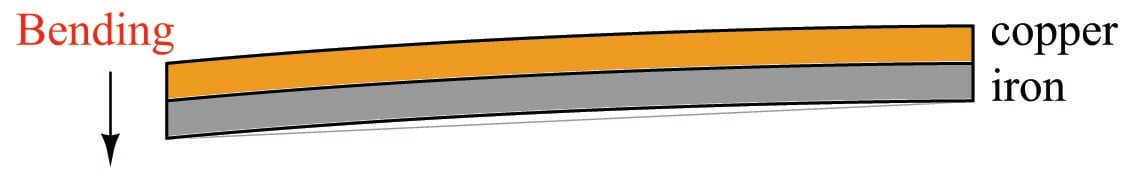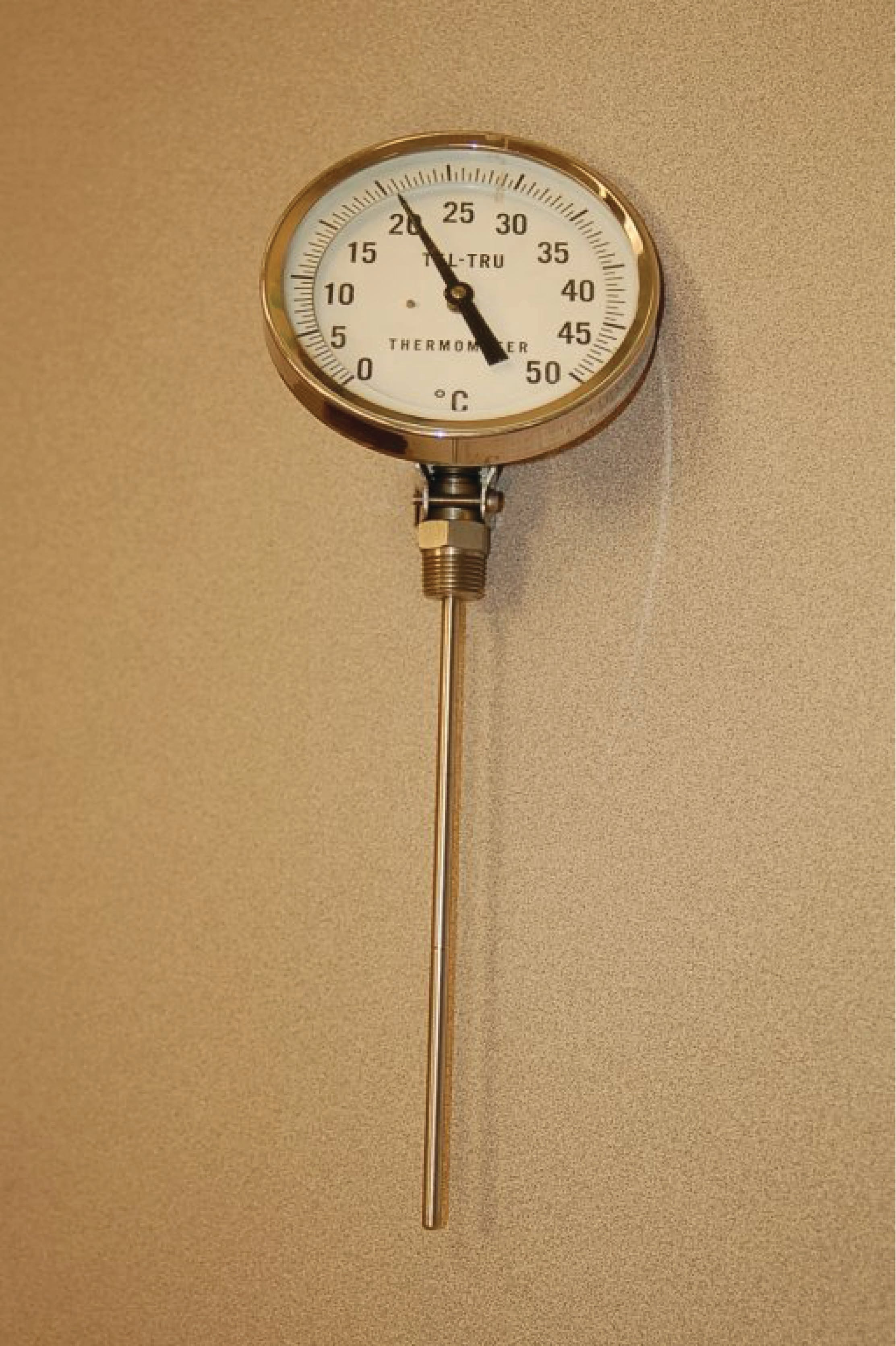Vol. Fluid Systems
Chapter Introduction to Continuous Temperature Measurement

# Bi-metal Temperature Sensors

Solids tend to expand when heated. The amount that a solid sample will expand with increased temperature depends on the size of the sample, the material it is made of, and the amount of temperature rise. The following formula relates linear expansion to temperature change:

$l = l_0 (1 + \alpha \Delta T)$

Where,

$$l$$ = Length of material after heating

$$l_0$$ = Original length of material

$$\alpha$$ = Coefficient of linear expansion

$$\Delta T$$ = Change in temperature

Here are some typical values of $$\alpha$$ for common metals:

• Aluminum = 25 $$\times$$ $$10^{-6}$$ per degree C
• Copper = 16.6 $$\times$$ $$10^{-6}$$ per degree C
• Iron = 12 $$\times$$ $$10^{-6}$$ per degree C
• Tin = 20 $$\times$$ $$10^{-6}$$ per degree C
• Titanium = 8.5 $$\times$$ $$10^{-6}$$ per degree C

As you can see, the values for $$\alpha$$ are quite small. This means the amount of expansion (or contraction) for modest temperature changes are almost too small to see unless the sample size ($$l_0$$) is huge. We can readily see the effects of thermal expansion in structures such as bridges, where expansion joints must be incorporated into the design to prevent serious problems due to changes in ambient temperature. However, for a sample the size of your hand the change in length from a cold day to a warm day will be microscopic.

One way to amplify the motion resulting from thermal expansion is to bond two strips of dissimilar metals together, such as copper and iron. If we were to take two equally-sized strips of copper and iron, lay them side-by-side, and then heat both of them to a higher temperature, we would see the copper strip lengthen slightly more than the iron strip:If we bond these two strips of metal together, this differential growth will result in a bending motion greatly exceeding the linear expansion. This device is called a bi-metal strip:This bending motion is significant enough to drive a pointer mechanism, activate an electromechanical switch, or perform any number of other mechanical tasks, making this a very simple and useful primary sensing element for temperature. Older home thermostats often used this principle to both indicate room temperature and to actuate electrical switches for controlling room temperature. Electric hot water heater units still use this type of device (usually in the form of a convex bi-metal disk) to sense over-temperature conditions and automatically shut off power to the heater if the water temperature exceeds a pre-set limit.

If a bi-metallic strip is twisted over a long length, it will tend to un-twist as it heats up. This twisting motion may be used to directly drive the needle of a temperature gauge. This is the operating principle of the temperature gauge shown in the following photograph:#### Lessons in Industrial Automation

Published under the terms and conditions of the Creative Commons Attribution 4.0 International Public License